﻿ Application of VIM Method for Nonlinear Porous Media Equations

### Application of VIM Method for Nonlinear Porous Media Equations

E. Abdolmaleki, S.A. YousefiOPEN ACCESSPEER-REVIEWED

## Application of VIM Method for Nonlinear Porous Media Equations

E. Abdolmaleki1,, S.A. Yousefi2

1Department of Applied Mathematics, Tonekabon Branch, Islamic Azad University, Tonekabon, Iran

2Department of Applied Mathematics, Shahid Beheshti University, Tehran, Iran

### Abstract

In this paper, we applied He’s variational iteration method (VIM) to solve nonlinear porous media equations. The main advantage of this method is the flexibility to give approximate solutions to nonlinear problems without linearization or discretization. The results show that this method is simple and effective.

### At a glance: Figures

1
Prev Next

• Abdolmaleki, E., and S.A. Yousefi. "Application of VIM Method for Nonlinear Porous Media Equations." American Journal of Numerical Analysis 2.1 (2014): 11-13.
• Abdolmaleki, E. , & Yousefi, S. (2014). Application of VIM Method for Nonlinear Porous Media Equations. American Journal of Numerical Analysis, 2(1), 11-13.
• Abdolmaleki, E., and S.A. Yousefi. "Application of VIM Method for Nonlinear Porous Media Equations." American Journal of Numerical Analysis 2, no. 1 (2014): 11-13.

 Import into BibTeX Import into EndNote Import into RefMan Import into RefWorks

### 1. Introduction

The porous media equations arise in the nonlinear problems of heat and mass transfer, combination theory and in biological systems. The nonlinear heat equation called the porous media equation is given in  as;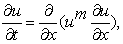(1)

where m is a rational number Eq. (1) is called a degenerate parabolic differential equation because the diffusion coefficient D (u) = um does not satisfy the condition for classical diffusion equations, D (u )> 0.

A discussion of the formulation of these models is given in [1, 2, 3]. Equations of the form (1) admit traveling-wave solutions u = u (Mx + Nt) where M and N are constants . The variational iteration method is a new method for solving nonlinear problems and was introduced by a Chinese mathematician, He [4, 5, 6, 7]. In  He, modified the general Lagrange multiplier method  and constructed an iterative sequence of functions which converges to the exact solution. The variational iteration method is used to address several problems, most of them well-known differential equations, which arise in several fields of physics and engineering. A criterion for convergence of the variational iteration method is introduced in  by means of the Banach fixed point theorem. Recently, many researchers have applied VIM method for different problems; see [10, 11] and reference there in.

In this paper we use the variational iteration method (VIM) for solving porous media equations in order to find the approximate solution.

The article is organized as follows. In section 2, we describe the basic formulation of the variational iteration method required for our subsequent development. Section 3 is devoted to the solution of Eq. (1) by using the variational iteration method. In section 4, we report our numerical findings and demonstrate the accuracy of the proposed scheme by considering numerical examples.

### 2. Variational Iteration Method

The variational iteration method is used in  for finding the minimum of a functional over the specified domain. Using this technique the solution of the problem is provided in a closed form while the mesh point techniques provide the approximation at mesh points only. In  the variational iteration method is employed to solve time-dependent reaction – diffusion equation which has special importance in engineering and sciences and constitutes a good model for many systems in various fields. This method is employed in  to solve the Klein-Gordon equation which is the relativistic version of the Schrodinger equation. which is used to describe spinless particles. Application of He’s variational iteration techniques to an inverse parabolic problem is described in . The variational iteration method and Adomian decomposition method are used and compared in , for solving a biological population equation. The main advantage of the two methods over the mesh points methods  is the fact that they do not require discretization of the variables. Furthermore, the variational iteration method overcome the difficulty arising in calculating the Adomian polynomials which is an important advantage over the Adomian decomposition method [17, 18].

The varietional iteration method is applied in  to find solution of an inverse problem for the semi-linear parabolic partial differential equation. The modified variational iteration method is used in  to solve a system of differential equations. The parabolic integro-differential equations arising in heat conduction in materials with memory is studied in  and the variational iteration method is used to find the solution of the problem the interested reader can see [22-29] for some other applications of the method.

Consider the following general nonlinear problem: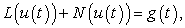(2)

where L is a linear operator, N is a nonlinear operator, and g(t) is a known analytical function. The variational iteration method constructs and iterative sequence called the correction functional as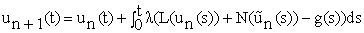(3)

where λ is the general Lagrange multiplier  which can be identified optimally via the variational theory [8, 22],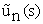is considered as the restricted variation , i.e.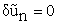, and the index n denotes the nth iteration.

### 3. The Approximate Solutions of the Porous Media Equation

Consider the porous media Eq. (1). Applying the variational iteration method Eq. (3) to Eq. (1) we have the following iteration sequence: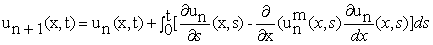According to the variational iteration method, we have correction functional: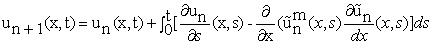Noticing that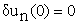, we get :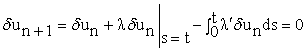Then, its stationary conditions can be obtained as: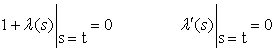The general Lagrange multiplier can therefore be readily identified: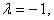As a result, we obtain the following iteration formula: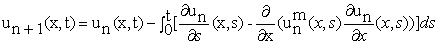### 4. Illustrative Examples

In this section we applied the method presented in this paper to two examples to show the efficiency of the approach.

4.1. Example 1

Let us take m = -1 in Eq. (1). We obtain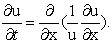(4)

The exact solution is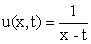.

Figure 1. Exact and approximate solutions for Example2 and u1 (x, t)

We applied the method presented in this paper and solved Eq. (4). The iteration formula for this example is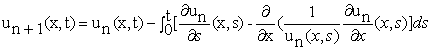Let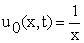. Applying the above iteration formula, we obtain: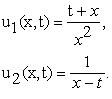Therefore, we obtain the exact solution.

4.2. Example 2

Let us take m = -4 / 3, Eq. (1) we obtain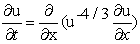(5)

The exact solution is u (x, t) = (2x - 3t)-3/4. Then the iteration formula is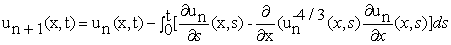(6)

Let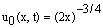. Applying the iteration formula (6), we have;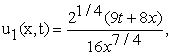And so on. The exact and approximate solutions for u1 (x, t) and u2 (x, t) are plotted in Figures. 1and 2. In each figure " green color " stand for the exact solution and " green color " stand for the approximate solutions.

### 5. Conclusion

In this paper the variational iteration method is used to solve the porous media equation. We described the method, used it on two test problems, and compared the results with their exact solutions in order to demonstrate the validity and applicability of the method. Moreover, only a small number of iterations are needed to obtain a satisfactory result the given numerical examples support this claim.

### References

  S. Pamuk, Solution of the porous media equation by Adomion’s decomposition method, phys.lett. A 344 (2005) 184-188.In article CrossRef  T. P. Witelski, J. Math. Biol. 35 (1997) 695.In article CrossRef  A. D. Polyanin, V. F. Zaitsev, Handbook of Nonlinear Partial Differential Equations, Chapman & Hall / CRC press, Boca Raton, 2004.In article  J. H. He, Variational iteration method for delay differential equations, commun. Nonlinear Sci. Number. Simul. 2 (4) (1997) 235-236.In article  J. H. He, Approximate analytical solution for seepage flow with fractional derivatives in porous media, Comput. Methods Appl. Mech. Engry. 167 (1998) 57-68.In article CrossRef  J. H. He, Approximate solution of nonlinear differential equation with convolution product nonlinearities, Comput. Methods Appl. Mech. Enggr. 167 (1998) 69-73.In article CrossRef  J. H. He, Variational iteration method-a kind of nonlinear analytical technique: some examples, Int. J. Non-linear Mech. 34 (1999) 699-708.In article CrossRef  M. Inokuti, et al., General use of the Lagrange multiplier in non-linear mathematical physics, in: S, Nemat-Nasser (Ed.), Variational Method in the Mechanics of solids, pergamon Press, Oxford. 1978, pp. 156-162.In article  M. Tatari, M. Dehghan, On the convergence of He’s variational iteration method, J. Comput. Appl. Math. 207 (2007) 121-128.In article CrossRef  G.C. Wu, D. Baleanuc, Variational iteration method for the Burgers’ flow with fractional derivatives-New Lagrange multipliers, 37 (2013), 6183-6190.In article  A. Maidia, J.P. Corriou, Open-loop optimal controller design using variational iteration method, Applied Mathematics and Computation,219 (2013) 8632-8645.In article CrossRef  M. Tatari. M. Dehghan, solution of problems in calclus of variations via He’s variational iteration method, phys. Lett. A362 (2007) 401-406.In article CrossRef  M. Dehghan, F. Shakeri, Application of He’s variational iteration method for solving the Cauchy reaction diffusion problem, J. Comput. Appl. Math. 214 (2008) 435-446.In article CrossRef  F. Shakeri, M. Dehghan, Numerical solution of the Klien-Gordon equation via He’s variational iteration Method, Nonlinear Dynam. 51 (2008) 89-97.In article CrossRef  M. Dehghan, M. Tatari, Identifying an unknown function in a parabolic equation with over specified data via He’s variational iteration method, chaos solitons Fractals 36 (2008) 157-166.In article CrossRef  M. Dehghan, F. shakeri, Numerical solution of a biological Population model using He’s variational iteration method, comput. Math. Appl. 54 (2007) 1197-1209.In article CrossRef  M. Dehghan, Finite difference procedures for solving a problem arising in modeling and design of certain optoelectronic devices, Math. Comput. Simulation 71 (2006) 16-30.In article CrossRef  M. shakourfar, M. Dehghan, on the numerical solution of nonlinear systems of volterla integro-differential equations with delay arguments, computing 82 (2008) 241-260.In article CrossRef  M. Dehghan, M. Shakourifar, A. Hamidi, The solution of linear and nonlinear systems of Volterra functional equations using Adomian-Pade technique, Chao, solitons and fractals 39 (2009) 2509-2521.In article CrossRef  M. Tatari, M. Dehghan, He’s variational iteration method for computing a control parameter in a semi-linear inverse parabolic equation, chaos, solitions and Fractals 33 (2007) 671-677.In article CrossRef  M. Tatari, M. Dehghan, Improvement of He’s variational iteration method for solving systems of differential equation s, computers and Mathematics whit Applications 58 (11-12) (2009) 2160-2166.In article  M. Dehghan, F. Shakeri, solution of paravolic integro-differential equations arising in heat conduction in materials with memory via He’s variational iteration technique, communication in Numerical Methods in Engineering (2008) (inpress).In article  S. Momani, S. Abuasad, Application of He’s variational iteration method to Helmholtz equation, chao solitons Fractals 27 (5) (2006) 1119-1123.In article  H. Tari, D. D. Ganji, M. Rostamian, Approximate solutions of K (2, 2), kdv and modified kdv and modified kdv equations by variabional iteration method, homotopy perturbation method and homotopy analysis method, Int. J. Nonlinear Sci. Numer. Simul. 8 (2) (2007) 203-210.In article CrossRef  S. Momani, Z. Odibat, Numerical comparison of methods for solving linear differential equations of fractional order, chao solution Fractals 31 (2007) 1238-1255.In article  Z. M. Odibat, S. Momani, Application of variational iteration method to Nonlinear differential equations of fractional order, Int. J. Nonlinear Sci. Numer. Simul. 7 (2006) 27-34.In article CrossRef  N. Bildik, A. Konuralp, The use of variational iteration method, differential transform method and Adomian decomposition method for solving different types of nonlinear partial differential equations, Int. J. Nonliner Sci. Numer. Simul. 7 (2006) 65-70.In article  J. H. He, X.H. Wu, Construction of solitary solution and compacton-like solution by variational iteration mebhod, chaos solitons Fractals 29 (2006) 108-113.In article CrossRef  N. H. Sweilam, M. M. Khader, Variational iteration method for one dimensional nonlinear the rmoelasticity, chaos solitions Fractals 32 (2007) 145-149.In article CrossRef  B. A. Finlayson, The Method of Weighted Residuals and Variational principles, Academic press, New York, 1972.In article  J. H. He, some asymptotic methods for strongly nonlinear equations, Int. J. Mod phys. B20 (10) (2006) 1141-1199.In article CrossRef